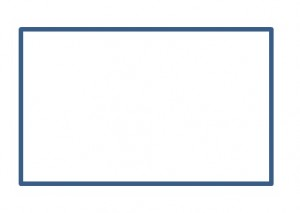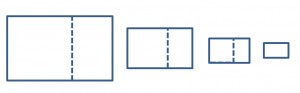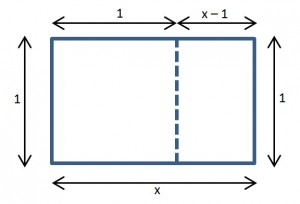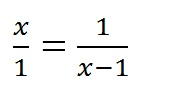# Rectangles and the Golden Ratio

These may all be digressions, but (as I reassure my students all the time), stay with me.  I’m heading somewhere with all of this.  For now, let’s just keep going.

Here is a rectangle:And, yes, in Your First Book of Shapes or its equivalent, that was the shape on the “Square” page.  But then in geometry class, you learned that the squares are a subset of the rectangles.  They just happen to have equal length and width.  Still, when I say “picture a rectangle”,  you probably don’t picture a square.  So I’ll try again.

Here is a rectangle:OK, that is surely a rectangle and not a square.  But is it what you picture when I say “rectangle”?  I’m guessing that my rectangle is skinnier than the one in your mind.  Some rectangles are more “rectangular-y” than others.  We could say they have higher “rectangularity”.  But is there a way to put a number on that?  Actually, there is.  We can use ratios.

Ratios are perfect for this job.  A ratio is a comparison using division.  To find the ratio of A to B, we calculate A÷B.  The answer tells us how many times bigger A is than B.

So let’s define the “Rectangularity” of a rectangle as the ratio of its length to its width.  In that case, my first rectangle had rectangularity of 1 which made it a square.  And my second rectangle had rectangularity of 12.  Its length was 12 times as great as its width.

We can keep calling this ratio “rectangularity”, a name that I made up, but in fact it already has a name: the length-to-width ratio of a rectangle is called its “aspect ratio”.    And in classical design, there is an aspect ratio that is considered ideal.  It just looks right: not too square-y, not too rectangular-y …just right.    It is called the Golden Ratio.  You can find tons of information about  it on-line so I won’t try to replicate it all here.  I just want to look at one interesting feature of a golden rectangle and show how we can use that feature to calculate the Golden Ratio.It looks nice.  But here’s the interesting property.

Slice off a square on one end…that little rectangle that remains is also a golden rectangle.  And yes, if you slice off the end square of this little rectangle, you get another even littler golden rectangle.Repeat as many times as you like to produce an endless stream of ever-smaller golden rectangles.Let’s start again.  Suppose the width of the rectangle = 1 and the length = x.Since the ratio  x/1=x, x must also be the value of the golden ratio.  But what is that value?

Well, for the little rectangle, the length is 1 and the width is x-1.  So that rectangle has an aspect ratio of 1/(x-1).  But that aspect ratio is the same as the aspect ratio of the bigger rectangle we started with.  We can write:To find the value of the golden ratio, we just have to solve that equation.  When you cross-multiply, you get:

x(x-1) = 1

You can distribute to get:

x2 – x = 1

But now what?  If you use trial and error with a calculator, you will soon find that the golden ratio is a little more than 1.6.  But if you want an exact solution, you need to know how to handle quadratic equations.

And that’s what I really want to talk about.  Quadratic equations pop up in physics.  Not every day, but occasionally.  And they usually can’t be factored.  (Neither can the one we have here.)  My students deal with them a variety of ways.  Some grab their TI89s and let the calculator do the work.   Some of them  use the quadratic formula.  Almost nobody “completes the square”.

I admit that I used to make fun of completing the square as a classic piece of cook-book math – all procedure, no understanding.  But I think I was wrong about that.  I wasn’t picturing the procedure geometrically.  And, as I have mentioned in a few other posts, it’s better with pictures.  I’ll explain in the next post.

## One thought on “Rectangles and the Golden Ratio”

1. A distinctive feature of this shape is that when a square section is removed, the remainder is another golden rectangle ; that is, with the same aspect ratio as the first. Square removal can be repeated infinitely, in which case corresponding corners of the squares form an infinite sequence of points on the golden spiral , the unique logarithmic spiral with this property.